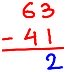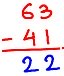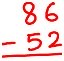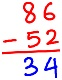# SUBTRACTION

##### Hello children, welcome back to class

SUBTOPIC: SUBTRACTION OF WHOLE NUMBERS GREATER THAN NINE

Numbers greater than 9 have more than 1 digits. Eg 15, 29, 125, etc

In subtracting 2-digit numbers we will subtract or minus a two-digit number from another two-digit number. To find the difference between the two numbers we need to subtract ‘ones from ones’ and ‘tens from tens’.

Examples

1. Subtract 41 from 63.

(i) First arrange the numbers vertically so that the tens’ place digits and ones’ place digits are lined up which means in simple one number should be written above the other number. Draw a line under the bottom number.

(ii) Subtract the digits in the ones place. Subtract (3 – 1 = 2). Place 2 in the ones column as shown.

(iii) Subtract the digits in the tens’ place. Subtract (6 – 4 = 2). Place 2 in the tens column as shown.

(iv) The difference of 63 – 41 is 22

2.ACTIVITY

1. 2 5 – 1 5 =
2. 4 8 – 1 2 =
3. 7 2 – 20 =
4. 1 8 – 6   =
5. 5 9 – 4 7 =
6. 6 3 – 4 1 =
7. 3 5 – 1 4 =

MORE ACTIVITYYour Opinion Matters! Quickly tell us how to improve your Learning Experience HERE